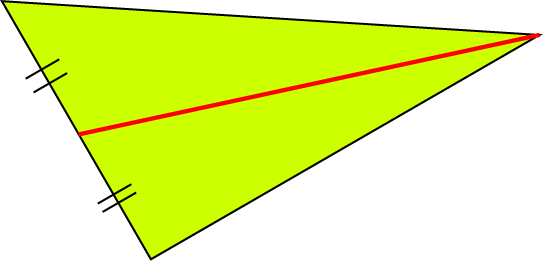# Definition of Median of a TriangleA median of a triangle is one of the three line segments that join each vertex (corner) to the midpoint of the opposite side of the triangle.

The three medians of a triangle all intersect (cross over) at a single point.

The place where the three medians intersect is a point called the centroid of the triangle.

### Description

The aim of this dictionary is to provide definitions to common mathematical terms. Students learn a new math skill every week at school, sometimes just before they start a new skill, if they want to look at what a specific term means, this is where this dictionary will become handy and a go-to guide for a student.

### Audience

Year 1 to Year 12 students

### Learning Objectives

Learn common math terms starting with letter M

Author: Subject Coach
You must be logged in as Student to ask a Question.